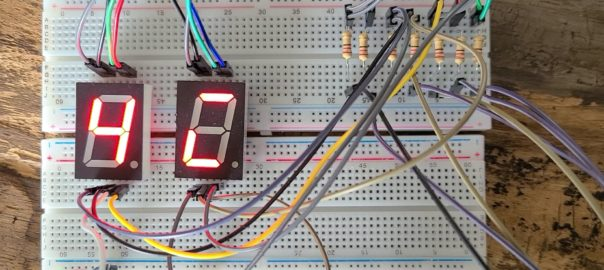# Daily Archives: January 10, 2021# Anode vs Cathode

When you deal with diode or transistor components such as LED, 7 Segment display, RGB LED, etc., you need to understand whether the component is common anode or common cathode, it will give you completely different result.

## 7 Segment Display

Let’s us the 7 Segment display as example.

All a – g & dp are connected to a ‘common’. When the common is common anode, we need to connect this to a high voltage, sometime 5V, so that current will be generated when a – g & dp is LOW. On the other hand, if the common is common ground, we need to connect this to a low voltage, most of the time is ground, so that current will be generated when a – g & dp is HIGH.

Common anode – the segment will be lit if the signal pins a – f & dp is LOW

Common cathode – the segment will be lit if the signal pins a -f & dp is HIGH.

As you can see from the above photos, left display is common cathode and right one is common anode. When we display a number 4 to the common cathode display, it will give the opposite result from the common anode. If you display a number 4 in the common anode device, it will give the same opposite result in common cathode device.

## Switching between 1 & 0

So, when you develop the program, you need to understand what device is going to use. Otherwise, you need to do some switching between 1 & 0, just like the program below.

```int start_pin = 2;

void setup() {
// put your setup code here, to run once:
int p;
for (p=start_pin; p<start_pin+7; p++){
pinMode(p, OUTPUT);
}
}

void loop() {
// put your main code here, to run repeatedly:
int i = 0;
int j = 0;
int number = 0;
int d = 0;
int segment = 0;
const int number_array[]={{1,1,1,1,1,1,0},
{0,1,1,0,0,0,0},
{1,1,0,1,1,0,1},
{1,1,1,1,0,0,1},
{0,1,1,0,0,1,1},
{1,0,1,1,0,1,1},
{1,0,1,1,1,1,1},
{1,1,1,0,0,0,0},
{1,1,1,1,1,1,1},
{1,1,1,0,0,1,1}
};

for (number=0;number<10;number++){
for (d=0;d<100;d++){
for (segment=0;segment<8;segment++){
digitalWrite(start_pin+segment, number_array[number][segment]);
}
delay(10);
}
}

for (number=0;number<10;number++){
for (d=0;d<100;d++){
for (segment=0;segment<8;segment++){
digitalWrite(start_pin+segment, not number_array[number][segment]);
}
delay(10);
}
}
}```

This program is developed with common cathode assumed, the number_array with 1 is on, 0 is off, i.e. 4 is 0110011. When apply the same pattern to the common anode device, it will look like ‘1001100’, the monster you see in those picture.

So, we add a ‘not’ to the line we highlighted in the code, it will give the opposite value to the pin, i.e. 1 -> 0, 0 -> 1. Thus, the data output of 4 will be changed from ‘0110011’ to ‘1001100’, it will be 4 in the common anode but a monster in common cathode.

## RGB LED

7 segment is quite easy to hand. But, take a look of RGB LED. I would prefer using common cathode RGB LED, because it is easy for me just follow the color code. But for common anode one, you need to do something like 255 – the color code as shown below, have a try!

```  analogWrite (red, 255 - 232); # Color code 232, 97, 0 (E86100)
analogWrite (green, 255 - 97);
analogWrite (blue, 255 - 0);
delay(1000);

analogWrite (red, 255 - 199); # Color code 199, 128, 35 (C78023)
analogWrite (green, 255 - 128);
analogWrite (blue, 255 - 35);
delay(1000);  ```